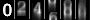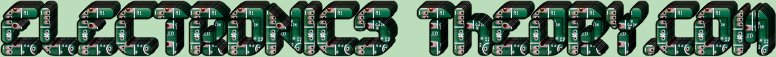# Enough is Enough !!

Ok, a human being can only be subjected to so much math before their head explodes. I don't know about you, but I've about reached my limit for now. So let's discuss something a little less math intensive.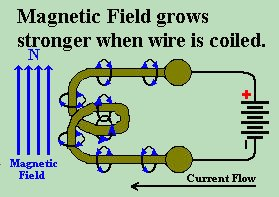We spoke earlier of the ability of a wire to produce a magnetic field when a current is flowing through it. We say that the current is INDUCING the magnetic field. We also stated that this inductance becomes stronger when the wire is wound up into a coil. But this is not the only factor which effects the amount of inductance within a coil. There are actually 4 factors which determine the inductance of a coil of wire: The number of turns in the coil. The material that the CORE of the coil is made of. The length of the coil. The diameter of the coil
NUMBER OF TURNS:
If we have two coils of identical length and diameter, but one has 4 turns of wire, while the second has 8 turns of wire, the coil with 8 turns will have more inductance than the one with 4 turns.
CORE MATERIAL:
A coil wrapped around an iron core will have a much greater inductance than one wound with nothing in the middle but air. This is because iron has a much higher PERMEABILITY than air.
Permiability is (roughly) the amount of magnetism absorbed by a material. Permiability of a vacuum (or free space) is said to be 1. Iron depends on its level of impurities. Pure iron would be on the order of 200,000, whereas carbon steel can be as low as 100. Ferrites can be 15-500.
LENGTH OF COIL:
If the coil has the same diameter, and the same number of turns, but is made longer (say, by stretching it out), the inductance of the coil will decrease.
DIAMETER OF COIL:
If the coil is the same length and number of turns, but smaller in diameter, the inductance will also be smaller.

While not important for this discussion, the basic Formula for Inductance is as follows:
L=p* (N*N) * A / l

Where
L = inductance in Henry (H)
p = permeability (Wb/A.m)
N = number of turns in the coil
A = area encircled by the coil
l = length of the coil(m)

We could go into the math and physics of why the coil increases or decreases with size, shape, number of turns, etc, but that is not the scope of this course. A basic general understanding of inductance is all you need at this point. So let us first explain what inductance is and how it works.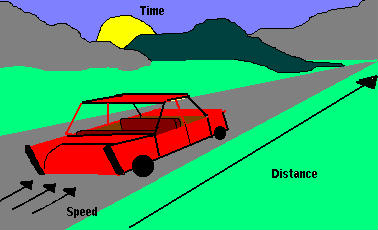When we first created our coil of wire, we were working with DC. Now we are learning a little about AC, and as you've found from the math.... the game changes some. When we attach a battery to our coil, it induces a magnetic field. This magnetic field does not, however, appear instantly, as one might believe.

You see, electricity, as we have found, moves at a given speed (the speed of light). It travels the distance of the wire, depending upon its length. Now we know that there is a special relationship between speed and distance, in that it takes TIME for something to travel a distance at a given speed.

Prior to our attaching the battery (we'll assume a 12 Volt battery for our discussion), the potential difference between the two ends of the wire is 0 Volts, and the current flowing through the wire is zero. As we attach the battery, the wire, which has resistance, enforces Ohm's Law. The Voltage (now 12V), divided by the resistance of the wire sets up a current within the wire. That current begins at one end of the wire, moving through the wire until it reaches the other end. During the time period of time that the current begins movement (0 speed), to the time it reaches its maximum speed, it is accelerating. Of course, when we disconnect the battery, we have an opposite effect, and for some time, the current is decelerating. The important point here is that the current speeds up and slows down within the coil whenever we attach or detach the voltage source.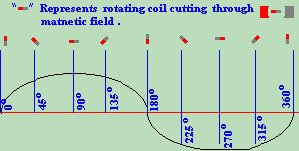Now for AC:
As we use an ALTERNATING current source, we know that the Voltage is in a constant state of change, fluctuating from 0 Volts, up to a maximum positive peak, back down to 0, continuing to a negative peak, then returning again to its starting point of 0 Volts. It takes TIME for this to happen. Now if we attach this fluctuating voltage source to a coil, the voltage is in a constant state of change. As the AC generator begins its upward curve, the voltage in the coil is 0 Volts. As the voltage reaches its positive peak, recall that it takes time for the electric current to flow, the voltage in the coil is beginning to rise, and a NORTH magnetic pole is beginning to be formed. As the coil reaches its peak of current, the alternator has rotated to its point of 0 Volts and is beginning to swing negative. (Can you predict what is coming?)

As the alternator swings negative, it forces current through the wire in the opposite direction. At the same time, the magnetic field in the coil attempts to collapse, by inducing its magnetic field into the wire in the same direction it was originally moving. The current that the magnetic field is producing, is in direct opposition to the current that the generator is now producing. Of course, the magnetic field is small, and has less staying power than the generator, which has force and momentum behind it. So the generator wins and pushes the current through the coil, now forming a SOUTH pole on the electromagnetic coil.So as the generator is swinging from positive to negative, the coil is swinging from North to South, expanding and collapsing between Voltage peaks. In addition, the coil is slightly out of phase (remember that word?) with the generator, and hence, is opposing the flow of electric current. Isn't that the definition of RESISTANCE? The coil RESISTS the flow of AC, but allows DC to pass freely. INDUCTANCE is defined as the opposition to any change in current flow. It is NOT the opposition to current flow, but the opposition to CHANGE of the flow. It is a form of light resistance caused by the collapsing magnetic field opposing the CHANGE in current within the coil.

Did we say that a coil acts like a resistor in some circumstances? Yup, sure did! Then it must follow Ohm's Law, huh? Yup, sure does! Not only does the wire itself have resistance, but the coil resists AC current flow. But Ohm's law doesn't EXACTLY apply in the same way, and for now, you've had enough math, so I won't throw that at you right away.

What you should consider, though, is that inductors can be act like resistors in parallel circuits. This means that you solve for inductance, the same way you solve for resistance in a series circuit.... you add the value of the inductors. Along the same lines, in a parallel circuit, you would "reciprocate the sum of the reciprocals" of individual inductances.
 (On The Following Indicator... PURPLE will indicate your current location) 1 2 3 4 5 6 7 8 9 10 11 12 13 14 15 16 17 18 19 20 21 22 23 24 25 26 27 28 29 30 31 32 33 34 35 36 37 38 39 40 41 42 43 44 45 46 47 48 49 50 51 52 53 54 55 56 57 58 59 60 61 62 63 64 65 66 67 68 69 70 71 72 73 74 75

[COURSE INDEX] [ELECTRONICS GLOSSARY] [HOME]Otherwise - please click to visit an advertiser so they know you saw their ad!

VISITORS: